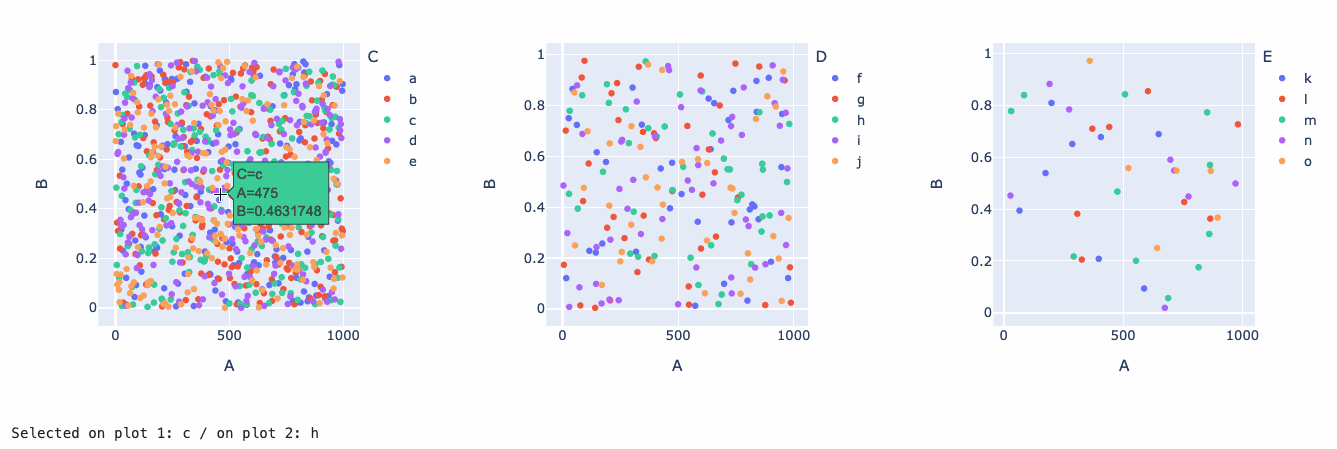# How do I link Plotly plots using click_data?

I have a use case where I want to display 3 plots.

First plot is a global view that shows categories. Second plot is a local view that shows each data point within the first plot selection. Third plot is a local view of that data point selected in the second plot.

I was using Plotly’s click_data param to make the second plot react to selection in the first plot:

``````plot_panel = pn.pane.Plotly(
xpl.plot.features_importance(),
config={"responsive": True}
)

@pn.depends(plot_panel.param.click_data)
def contribution_plot(click_data):
if click_data:
contribution_pane =  pn.pane.Plotly(
xpl.plot.contribution_plot(click_data['points']['label']),
config={"responsive": True}
)
else:
contribution_pane = pn.pane.Plotly(
xpl.plot.contribution_plot(xpl.features_imp.idxmax()),
config={"responsive": True}
)
return contribution_pane

material.main.append(
pn.Row(
pn.Column(
),
pn.Column(
pn.Tabs(
('Smart Explainer',
pn.Column(
plot_panel,
contribution_plot
)
)
)
)
)
)
``````

But, I’m struggling to do the same for the third plot, where the click_data depends on contribution_pane.param.click_data, since contribution_pane is defined during the function call.

FYI: this is the same functionality that https://shapash-demo2.ossbymaif.fr/ does. I’m just experimenting with panel to display the same features.

You could try something like that. A good part of the code is setting up the data, you can ignore that part and adapt it to your data structure. The approach I’ve used is to first create Plotly panes (I’ve initialized them, but they could just be empty) and add callbacks decorated with `@pn.depends(plotly_pane.param.click_data, watch=True)` that update the `.object` attribute of each pane, on a `click_data` event. I’ve also created a very simple state object, `selected`, to keep track of what is selected on each plot. I could also have used `global` variables, but ultimately if I’d have to keep more track of the state of the app I’d just wrap the whole thing is one or multiple classes. Hope this helps!

``````import numpy as np
import pandas as pd
import panel as pn
import plotly.express as px

pn.extension('plotly')

cats_c = list('abcde')
cats_d = list('fghij')
cats_e = list('klmno')
nb = 1000

df = pd.DataFrame({
'A': np.arange(nb),
'B': np.random.rand(nb),
'C': np.random.choice(cats_c, nb),
'D': np.random.choice(cats_d, nb),
'E': np.random.choice(cats_e, nb)
})
df = df.sort_values(['C', 'D', 'E'])

def create_fig(df, color, query=None):
if query:
df = df.query(query)
return px.scatter(df, x="A", y="B", color=color)

plot_panel1 = pn.pane.Plotly(create_fig(df, 'C'), width=400, height=400)
plot_panel2 = pn.pane.Plotly(create_fig(df, 'D', f'C == "{cats_c}"'), width=400, height=400)
plot_panel3 = pn.pane.Plotly(create_fig(df, 'E', f'C == "{cats_c}" and D == "{cats_d}"'), width=400, height=400)

class selected:
on1 = cats_c
on2 = cats_d

@pn.depends(plot_panel1.param.click_data, plot_panel2.param.click_data)
def string_hello_world(*args):
return pn.pane.Str(f'Selected on plot 1: {selected.on1} / on plot 2: {selected.on2}')

@pn.depends(plot_panel1.param.click_data, watch=True)
def _update_after_click_on_1(click_data):
# e.g. {‘points’: [{‘curveNumber’: 3, ‘pointNumber’: 5, ‘pointIndex’: 5, ‘x’: 74, ‘y’: 0.6295433958355939}]}
curve_index = click_data['points']['curveNumber']
selected.on1 = cats_c[curve_index]
plot_panel2.object = create_fig(df, 'D', f'C == "{selected.on1}"')

@pn.depends(plot_panel2.param.click_data, watch=True)
def _update_after_click_on_2(click_data):
# e.g. {‘points’: [{‘curveNumber’: 3, ‘pointNumber’: 5, ‘pointIndex’: 5, ‘x’: 74, ‘y’: 0.6295433958355939}]}
curve_index = click_data['points']['curveNumber']
selected.on2 = cats_d[curve_index]
plot_panel3.object = create_fig(df, 'E', f'C == "{selected.on1}" and D == "{selected.on2}"')

pn.Column(
pn.Row(plot_panel1, plot_panel2, plot_panel3),
string_hello_world,
)
``````1 Like